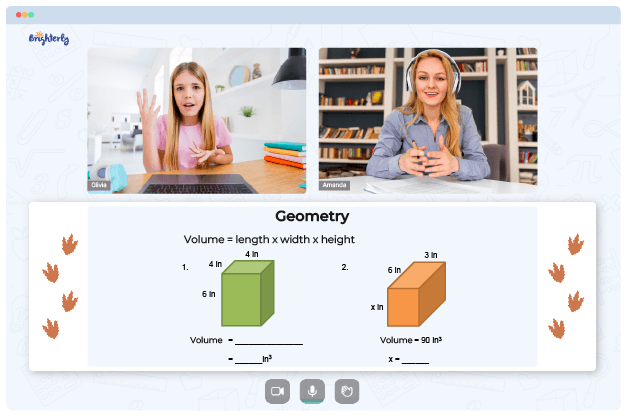# Types Of Triangles Worksheets

There are several types of triangles and they are classified based on their sides and angles. In this types of triangles worksheet, students will learn about the different types of triangles and how to identify them. The answer key identifying triangles worksheet answers will help student to solve related problems.

## What are the Types of Triangles?

The following are the different types of triangles:

Scalene triangles: Triangles in which all three sides have different lengths and all three angles have different measures.

Isosceles triangles: Triangles in which two sides have the same length and two angles have the same measure.

Equilateral triangles: Triangles in which all three sides have the same length and all three angles have the same measure (60 degrees).

Right triangles: Triangles in which one angle measures exactly 90 degrees.

Math for Kids

Is Your Child Struggling With Math?
1:1 Online Math TutoringAcute triangles: Triangles in which all three angles measure less than 90 degrees.

Obtuse triangles: Triangles in which one angle measures more than 90 degrees.

45-45-90 triangles: Right triangles in which the angles are 45 degrees, 45 degrees, and 90 degrees.

30-60-90 triangles: Right triangles in which the angles are 30 degrees, 60 degrees, and 90 degrees.### Types Of Triangles Worksheets PDF

Types Of Triangles Worksheet### Types Of Triangles Worksheets PDF### Types Of Triangles Worksheets PDF

Identifying Triangles Worksheet### Types Of Triangles Worksheets PDF

Our types of triangles worksheet pdf is available to download for free. You can also have access to our identifying triangles worksheet and identifying triangles worksheet answers pdf to solve problems on the different types of triangles. The types of triangles and identify triangles worksheet are easy to understand and the instructions are simplified for kids.

Need help with Geometry?• Does your child struggle to master geometry lessons?
• Try learning with an online tutor.

Is your child having trouble grasping the concept of geometry? An online tutor could provide the necessary assistance.